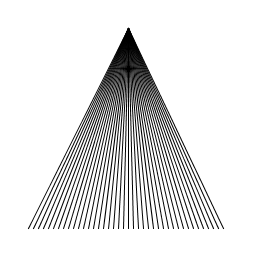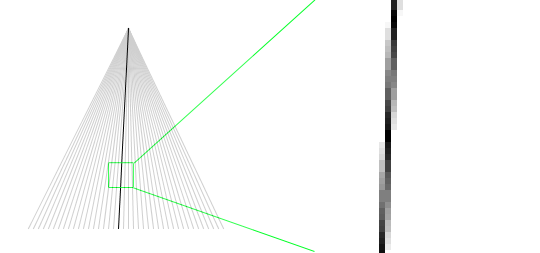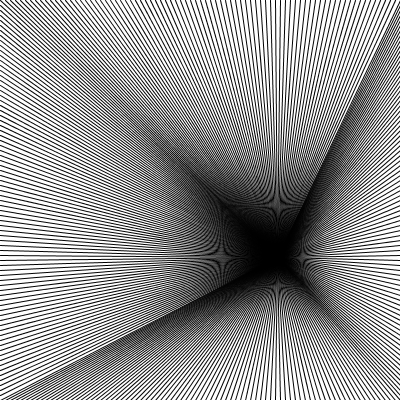## Moiré Loops in Java (Processing)

This tutorial loosley follows what we cover in our Intro to Java classes, and is a great example of what we'll cover in the early days of the class.

### Moiré Patterns

Here's an interesting application of loops - creating Moiré patterns (Wikipedia). A Moiré pattern is a pattern that is created as a visual artifact from drawing a lot of lines close together, like this:This is a very simple triangle made by only drawing straight lines from its top to its base. So, how did that diamond pattern get there? The secondary Moiré pattern is a result of the fact that we are drawing to a computer screen - a fixed grid of pixels, where we can only approximate a straight line. This approximation is achieved by filling in the pixels with varying amounts of color, like this:This shows one line in the triangle - at its actual size, and magnified 6x. If you lean back from your computer, and squint your eyes, then the line on the right will look like a straight line. This process of using shades of color to create an approximation of a line is called anti-aliasing. If you were to draw the same triangle on a piece of paper using a ruler to keep your lines perfectly straight, the Moiré pattern would never show up.

### Code

So, what does the code look like for creating the above pattern?

``````void setup()
{
size( 400, 400 );
}

void draw()
{
background( 255 );

noFill();
stroke( 0 );

for ( int x = 100; x < width - 100; x = x + 5 ) {
line( width / 2, 100, x, height - 100 );
}
}``````

This is a fairly simple `for` loop that uses the variable `x` to iterate along the base of the triangle, 5 pixels apart. In this example, the triangle is drawn 100 pixels in from the edges of the canvas, so its top is at `(width / 2, 100)` or `(200, 100)`, the bottom left point is `(100, height - 100)` or `(100, 300)`, and the bottom right point is at `(width - 100, height - 100)` or `(300, 300)`. Why not just use the fixed numbers, instead of calculating the points using the `width` and `height` special variables? Because this way, we can change the size of the canvas, and our code will just work with the new dimensions. Try it yourself by changing `size(400, 400)` to something else.

### A Moiré BoxSimilar to the triangle in how it's constructed, this draws lines to each edge of the canvas:

``````void setup()
{
size( 400, 400 );
}

void draw()
{
background( 255 );
noFill();
stroke( 0 );

// loop from left to right, drawing lines to the top of the canvas:
for ( int x = 0; x <= width; x = x + 5 ) {
line( mouseX, mouseY, x, 0 );
}

// loop from left to right, drawing lines to the bottom of the canvas:
for ( int x = 0; x <= width; x = x + 5 ) {
line( mouseX, mouseY, x, height );
}

// loop from top to bottom, drawing lines to the left side of the canvas:
for ( int y = 5; y < height; y = y + 5 ) {
line( mouseX, mouseY, 0, y );
}

// loop from top to bottom, drawing lines to the right side of the canvas:
for ( int y = 5; y < height; y = y + 5 ) {
line( mouseX, mouseY, width, y );
}
}``````

This version is fun to play with because it is using the current mouse position, `(mouseX, mouseY)`, as the starting point for all the lines. One thing you may have noticed is that there are four loops - one for each of the sides of the canvas. This is fine because it helps us think about the steps required to create the sketch, and makes the code quite readable - but we can optimize things a little. The two `for` loops that loop over x are exactly the same:

``````	// loop from left to right, drawing lines to the top of the canvas:
for ( int x = 0; x <= width; x = x + 5 ) {``````
``````	// loop from left to right, drawing lines to the bottom of the canvas:
for ( int x = 0; x <= width; x = x + 5 ) {``````

We can combine these two loops into one, like this:

``````	// loop from left to right:
for ( int x = 0; x <= width; x = x + 5 ) {
line( mouseX, mouseY, x, 0 ); // draw line to the top
line( mouseX, mouseY, x, height ); // draw line to the bottom
}``````

If we did the same for the two `for` loops that iterate over `y`, then the modified `draw()` function would look like this:

``````void draw()
{
background( 255 );
noFill();
stroke( 0 );

// loop from left to right:
for ( int x = 0; x <= width; x = x + 5 ) {
line( mouseX, mouseY, x, 0 ); // draw line to the top
line( mouseX, mouseY, x, height ); // draw line to the bottom
}

// loop from top to bottom::
for ( int y = 5; y < height; y = y + 5 ) {
line( mouseX, mouseY, 0, y ); // draw line to the left
line( mouseX, mouseY, width, y ); // draw line to the right
}
}``````

Try it out here.

### A More Dynamic Moiré Box

To see different aspects of the Moiré pattern, you can change the distance between the lines. This best achieved by introducing a variable - let's call it `increment`. Declare it at the top of your sketch, and use it wherever you see the number 5. Like this (modified lines are highlighted):

``````int increment = 5;

void setup()
{
size( 400, 400 );
}

void draw()
{
background( 255 );
noFill();
stroke( 0 );

// loop from left to right:
for ( int x = 0; x <= width; x = x + increment ) {
line( mouseX, mouseY, x, 0 ); // draw line to the top
line( mouseX, mouseY, x, height ); // draw line to the bottom
}

// loop from top to bottom::
for ( int y = increment; y < height; y = y + increment ) {
line( mouseX, mouseY, 0, y ); // draw line to the left
line( mouseX, mouseY, width, y ); // draw line to the right
}
}``````

Then, we can add a `mousePressed()` function (at the bottom of your sketch) to change the value of `increment` when you press the up or down arrow keys:

``````void keyPressed()
{
// make the lines further apart when the up arrow pressed:
if ( keyCode == UP ) {
increment++; // shortcut for increment = increment + 1;
}

// make the lines closer together when the down arrow pressed:
//  (but don't let increment get smaller than 1 - that would be a problem...)
else if ( keyCode == DOWN && increment > 1 ) {
increment--; // shortcut for increment = increment - 1;
}
}``````

There a few things to note here that might be new:

• `keyCode` is a built-in special variable that holds the value of the key that was pressed
• `UP` and `DOWN` are built-in constants for the up and down arrow keys
• the `&&` operator means and - so in english, that if statement (line 10) would read: "if the user pressed the down arrow key and the space between lines is more than 1." What do you think would happen if we let the value of `increment` go below 1?

You can try this new modified sketch, and see the full source code with keyboard control here.

### More Moiré - Two Circles

Moiré patterns are also created when two sets of lines overlap.  Here's an example with two circles:This is the code to create these overlapping circles:

``````void setup()
{
size( 600, 400 );
}

void draw()
{
background( 0 );

noFill();
stroke( 255 );

int oneThirdWidth = width / 3;
int twoThirdsWidth = 2 * width / 3;

// draw the set of concentric circles on the left:
for( int n = 0; n < twoThirdsWidth; n = n + 10 ) {
ellipse( oneThirdWidth, height / 2, n, n );
}

// draw the set of concentric circles on the right:
for( int n = 0; n < twoThirdsWidth; n = n + 10 ) {
ellipse( twoThirdsWidth, height / 2, n, n );
}
}``````

See if you can modify the sketch so that you can control the space between the concentric circles, or change the stroke weight, or how much they overlap.